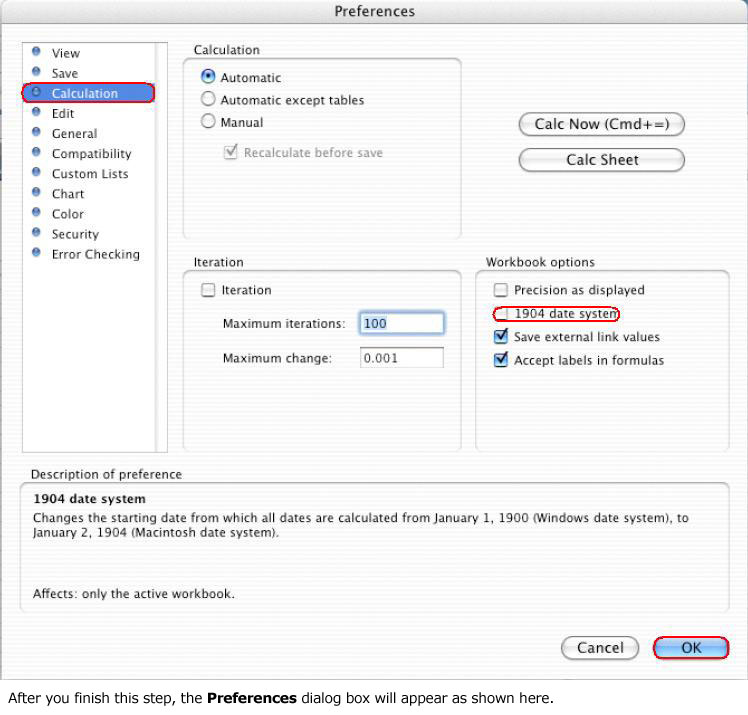# Excel中日期和时间函数如何使用

Excel 将所有日期存储为整数，将所有时间存储为小数。有了此系统，Excel 可以像处理任何其他数字一样对日期和时间进行加、减或比较操作，而且所有的日期都可以使用此系统进行处理。

### TODAY () 函数和 NOW () 函数

TODAY 函数根据您的系统时钟返回今天日期的序数，不包括时间。NOW 函数返回今天日期的序数，包括时间。

#### 如何根据序数比较日期

`=IF(NOW()=DATEVALUE("10/1/92"),TRUE,FALSE)`

`=IF(TODAY()=DATEVALUE("10/1/92"),TRUE,FALSE)`

### 如何使用日期公式

#### 如何确定今天与未来某个日期之间相隔的天数

`="mm/dd/yy"-NOW()`

#### 如何确定两个日期之间相隔的天数、月数和年数

1. 创建一个新工作簿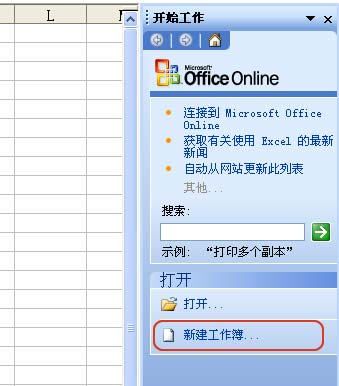2. 在新建工作簿工作栏上，点击空白工作簿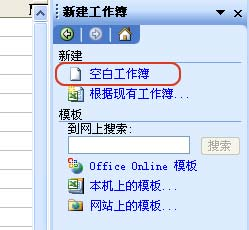3. 在工作簿中键入以下数据：
```A1：    03/25/94
A2：    05/01/98```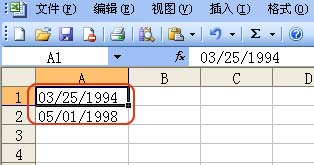4. 在单元格 D1 中键入以下公式：
```=YEAR(A2)-YEAR(A1)-IF(OR(MONTH(A2)<MONTH(A1),AND(MONTH(A2)=MONTH(A1),
DAY(A2)<DAY(A1))),1,0)&" years, "&MONTH(A2)-MONTH(A1)+IF(AND(MONTH(A2)
<=MONTH(A1),DAY(A2)<DAY(A1)),11,IF(AND(MONTH(A2)<MONTH(A1),DAY(A2)
>=DAY(A1)),12,IF(AND(MONTH(A2)>MONTH(A1),DAY(A2)<DAY(A1)),-1)))&" months,
"&A2-DATE(YEAR(A2),MONTH(A2)-IF(DAY(A2)<DAY(A1),1,0),DAY(A1))&" days"```
展开这个图片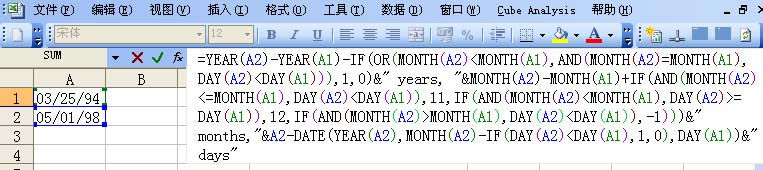注意：复制和粘贴该公式时，一定不能包含换行符，否则该公式无效。

`4 years, 1 months, 6 days`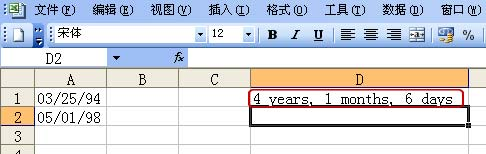```时间部分                     公式
------------------------------------------------------------------------

DAY(A1)),12,IF(AND(MONTH(A2)>MONTH(A1),
DAY(A2)<DAY(A1)),-1)))&" months"```
```两个日期之间          =YEAR(A2)-YEAR(A1)-IF(OR(MONTH(A2)<

DAY(A2)<DAY(A1))),1,0)&" years"```

#### 如何确定两个日期之间相隔的工作日数

1. 创建一个新工作簿。2. 在新建工作簿工作栏上，点击空白工作簿3. 在工作簿中键入以下数据：
```A1：    03/25/94
A2：    05/01/98```4. 在单元格 D1 中，键入以下公式：
`=NETWORKDAYS(A1,A2)`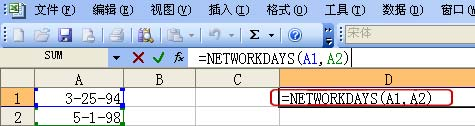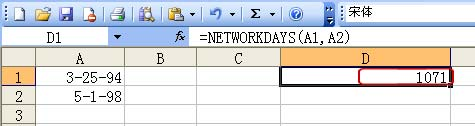#### 如何递增日期

`=DATE(YEAR(reference)+value1,MONTH(reference)+value2,DAY(reference)+value3)`

```=DATE(YEAR(DATEVALUE("6/20/96")),MONTH(DATEVALUE("6/20/96"))+1,
DAY(DATEVALUE("6/20/96")))```

### 如何使用时间公式

#### 如何计算经过的时间

```A1：开始时间         B1：结束时间     C1：差        D1：差
（常规）        (h:mm)
A2：06:30:00 AM    B2：7:15 PM    C2：0.53125  D2： 12:45```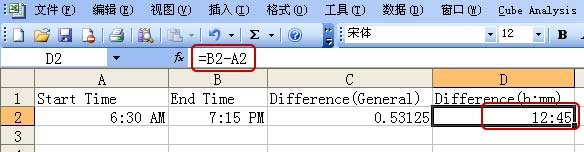```A1：开始时间        B1：结束时间     C1：差            D1：差
（常规）          (h:mm)
A2：7:45 PM       B2：10:30 AM    C2：0.61458333  D2： 14:45```

`=B2-A2+IF(A2>B2,1)`

`[h]:mm`

#### 如何将日期转换成对应的小数

`=Time*24`

`=A1*24`

`=(Time-INT(Time))*24`

`=(A1-INT(A1))*24`

#### 如何将小数转换成对应的日期

`=Time/24`

`=A1/24`

### 如何在 Microsoft Excel for the Macintosh 和 Microsoft Excel for Windows 之间传送文件

1. 工具菜单上，单击选项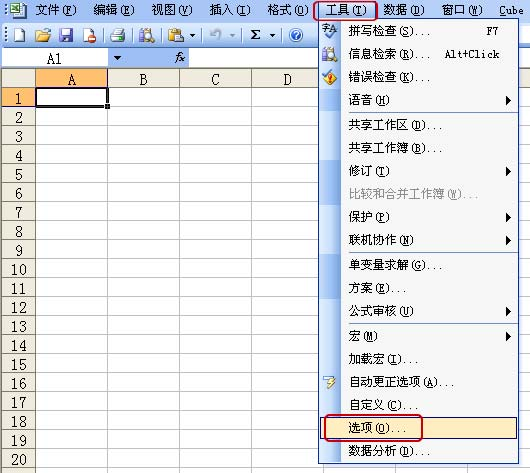2. 单击计算选项卡，然后选中“1904 年日期系统”复选框。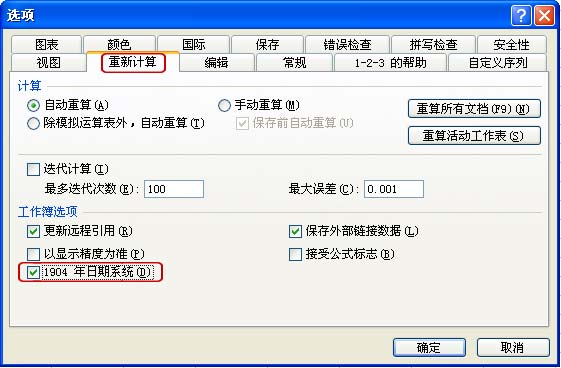1. 工具菜单上，单击首选项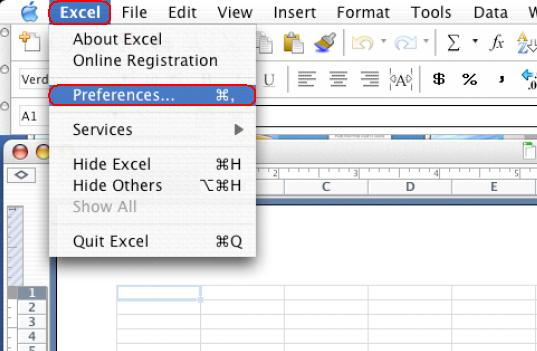2. 单击计算选项卡，然后清除“1904 年日期系统”复选框。
展开这个图片